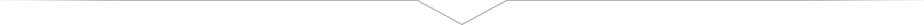### Calculations for gas struts

In order to determine the correct strut for an application, it is important to calculate the required force. The calculation is not difficult, but must be done accurately. In order to calculate the required force of a gas strut, it is important to know how it will be applied. There are two different applications:

• Applying a gas strut to a hatch. In a situation like this, the construction will open from a horizontal position to a vertical one.
• Applying a gas strut to a flap. In a situation like this, the construction will open from a vertical position to a horizontal one.
• Gas traction strut applied in a situation where it is not possible to fit a gas pressure strut.

In order to calculate the approximate Newton pressure of a gas strut, only a few more variables are required, such as:

• Starting angle
• The lever arm (Length from pivot point to end of flap/hatch)
• Distance from pivot point to centre of gravity
• Desired opening angle
• Thickness flap/hatch
• The weight force of the flap or hatch in kilos
• The number of gas struts

### Further gas strut calculation criteria

Once you have calculated the required force, you can start selecting other strut criteria. One of them is the choice of material. Not all materials are suitable for the environment in which your specific gas strut will be applied. Temperature, weight and, for example, corrosion resistance may limit your options.

Having a clear idea beforehand of the gas strut application could prevent many problems. Using suitable gas struts for the application in question means they will be subject to less wear and tear and will not fail. You will also avoid having to use an unnecessarily heavy and expensive gas strut.

Needless to say, we would be more than happy to advise you about things like the choice of material. You can also contact SpringMasters for advice pertaining to the calculation of the required force.

X

Gas Strut for hatch situation

Gas Strut for flap situation

Traction Strut situationStarting angleH=length hatch/flapS= distance to center of gravity

R= opening angleT= thickness of hatch/flapG=WeightNumber of Gas Struts

Material version

SpringMasters is free to determine the attachment points

Note